# Binary Tree Traversal Techniques : Inorder-Preorder-Postorder-Levelorder A+ A  A-

Binary Tree Traversal Techniques:

A tree traversal is a method of visiting every node in the tree. By visit, we mean that some type of operation is performed. For example, you may wish to print the contents of the nodes.

There are four common ways to traverse a binary tree:d

1. Preorder
2. Inorder
3. Postorder
4. Level order

In the first three traversal methods, the left subtree of a node is traversed before the right subtree. The difference among them comes from the difference in the time at which a root node is visited.

Recursive Traversal Algorithms:

Inorder Traversal:

In the case of inorder traversal, the root of each subtree is visited after its left subtree has been traversed but before the traversal of its right subtree begins. The steps for traversing a binary tree in inorder traversal are:

1. Visit the left subtree, using inorder.
2. Visit the root.
3. Visit the right subtree, using inorder.

Preorder Traversal:

In a preorder traversal, each root node is visited before its left and right subtrees are traversed. Preorder search is also called backtracking. The steps for traversing a binary tree in preorder traversal are:

1. Visit the root.
2. Visit the left subtree, using preorder.
3. Visit the right subtree, using preorder.

Postorder Traversal:

In a postorder traversal, each root is visited after its left and right subtrees have been traversed. The steps for traversing a binary tree in postorder traversal are:

1. Visit the left subtree, using postorder.
2. Visit the right subtree, using postorder
3. Visit the root.

Level order Traversal:

In a level order traversal, the nodes are visited level by level starting from the root, and going from left to right. The level order traversal requires a queue data structure. So, it is not possible to develop a recursive procedure to traverse the binary tree in level order. This is nothing but a breadth first search technique.

Example 1:

Traverse the following binary tree in pre, post, inorder and level order.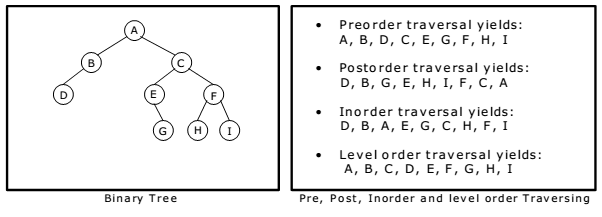Non Recursive Traversal Algorithms:

At first glance, it appears that we would always want to use the flat traversal functions
since they use less stack space. But the flat versions are not necessarily better. For
instance, some overhead is associated with the use of an explicit stack, which may
negate the savings we gain from storing only node pointers. Use of the implicit function
call stack may actually be faster due to special machine instructions that can be used.

Inorder Traversal:
Initially push zero onto stack and then set root as vertex. Then repeat the following
steps until the stack is empty:
1. Proceed down the left most path rooted at vertex, pushing each vertex onto the
stack and stop when there is no left son of vertex.
2. Pop and process the nodes on stack if zero is popped then exit. If a vertex with
right son exists, then set right son of vertex as current vertex and return to step
one.

Preorder Traversal:
Initially push zero onto stack and then set root as vertex. Then repeat the following
steps until the stack is empty:
1. Proceed down the left most path by pushing the right son of vertex onto stack, if
any and process each vertex. The traversing ends after a vertex with no left
child exists.
2. Pop the vertex from stack, if vertex ≠ 0 then return to step one otherwise exit.

Postorder Traversal:
Initially push zero onto stack and then set root as vertex. Then repeat the following
steps until the stack is empty:
1. Proceed down the left most path rooted at vertex. At each vertex of path push
vertex on to stack and if vertex has a right son push –(right son of vertex) onto
stack.
2. Pop and process the positive nodes (left nodes). If zero is popped then exit. If a
negative node is popped, then ignore the sign and return to step one.

Example :
Traverse the following binary tree in pre, post and inorder using non-recursive
traversing algorithm.Inorder Traversal:
Initially push zero onto stack and then set root as vertex. Then repeat the following
steps until the stack is empty:
1. Proceed down the left most path rooted at vertex, pushing each vertex onto the
stack and stop when there is no left son of vertex.
2. Pop and process the nodes on stack if zero is popped then exit. If a vertex with
right son exists, then set right son of vertex as current vertex and return to step
one.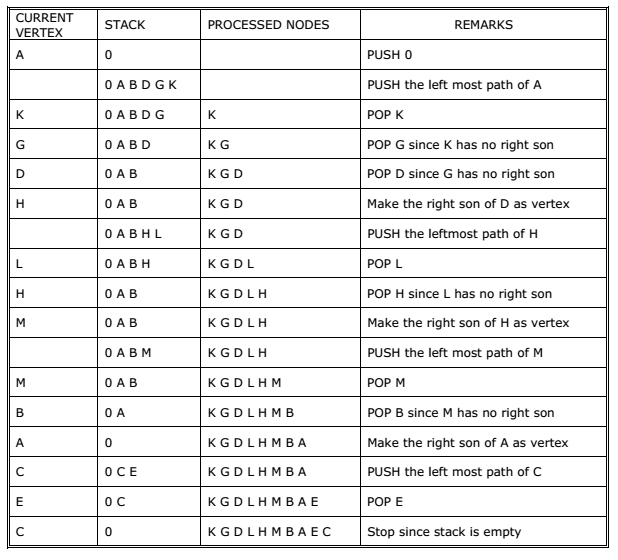Postorder Traversal:
Initially push zero onto stack and then set root as vertex. Then repeat the following
steps until the stack is empty:
1. Proceed down the left most path rooted at vertex. At each vertex of path push
vertex on to stack and if vertex has a right son push –(right son of vertex) onto
stack.
2. Pop and process the positive nodes (left nodes). If zero is popped then exit. If a
negative node is popped, then ignore the sign and return to step one.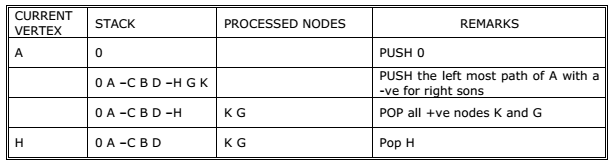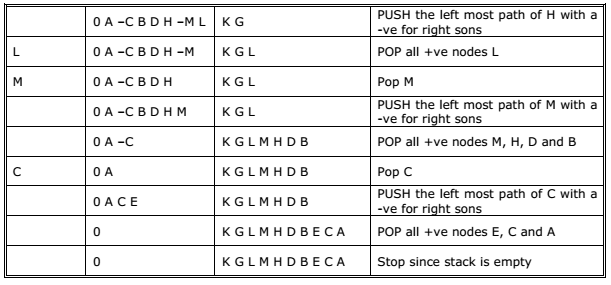Preorder Traversal:
Initially push zero onto stack and then set root as vertex. Then repeat the following
steps until the stack is empty:
1. Proceed down the left most path by pushing the right son of vertex onto stack, if
any and process each vertex. The traversing ends after a vertex with no left
child exists.
2. Pop the vertex from stack, if vertex ≠ 0 then return to step one otherwise exit.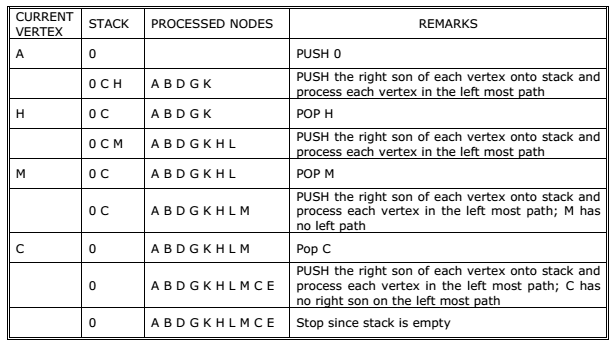Try Now – Data Structure MCQs
Practice Now – Data Structure : Binary Trees MCQ Based Online Test